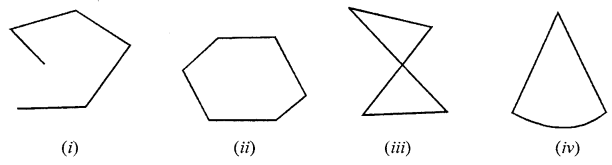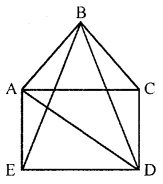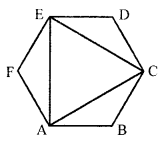## ML Aggarwal Class 6 Solutions for ICSE Maths Chapter 11 Understanding Symmetrical Shapes Ex 11.5

Question 1.
State whether the following statements are true (T) or false (F):
(i) Each angle of a rectangle is a right angle.
(ii) The opposite sides of a rectangle are equal in length.
(iii) The diagonals of a square are perpendicular to one another.
(iv) All sides of a rhombus are equal in length.
(v) All sides of a parallelogram are equal in length.
(vi) The opposite sides of a trapezium are parallel.
(vii) The diagonal of a parallelogram are equal.
Solution:
(i) True
(ii) True
(iii) True
(iv) False
(v) False
(vi) False
(vii) False

Question 2.
Examine whether the following figures are polygons. Give reasons.Solution:
(i) Not a polygon, because it is not a closed curve.
(ii) Polygon, because it is a simple closed curve made up entirely of line segments.
(iii) Not a polygon, because it is not a simple curve.
(iv) Not a polygon, because it is not made up of entirely line segments.

Question 3.
Name each of the following polygons:Solution:
(i) Pentagon
(iii) Hexagon
(iv) Octagon

Question 4.
Draw a rough sketch of a pentagon and draw its diagonals.
Solution:ABCDE is required pentagon.
Its diagonals are : AC, AD , BE, BD.

Question 5.
Draw a rough sketch of a regular hexagon. Connecting three of its vertices draw:
(i) an isosceles triangle
(ii) a light angled triangle.
Solution:
The triangle ACE obtained on joining its three vertices A, C and E is an equilateral triangle.Question 6.
Can you identify the regular quadrilateral?
Solution: